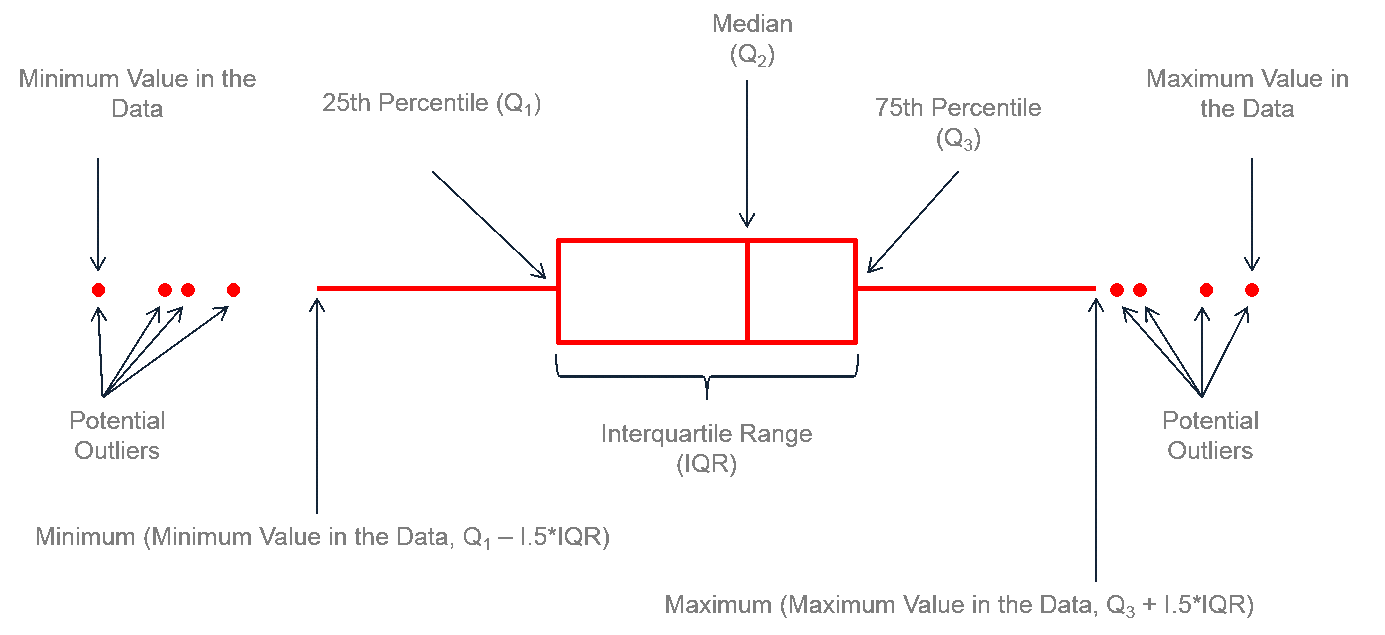A boxplot gives a nice summary of one or more numeric variables. A boxplot is composed of several elements:

• The line that divides the box into 2 parts represents the median of the data. If the median is 10, it means that there are the same number of data points below and above 10.
• The ends of the box shows the upper (Q3) and lower (Q1) quartiles. If the third quartile is 15, it means that 75% of the observation are lower than 15.
• The difference between Quartiles 1 and 3 is called the interquartile range (IQR)
• The extreme line shows Q3+1.5xIQR to Q1-1.5xIQR (the highest and lowest value excluding outliers).
• Dots (or other markers) beyond the extreme line shows potntial outliers.

Here is a diagram showing the boxplot anatomy:Anatomy of a boxplot (image source)

A boxplot can summarize the distribution of a numeric variable for several groups. The problem is that summarizing also means losing information, and that can be a pitfall. If we consider the boxplot below, it is easy to conclude that group `C` has a higher value than the others. However, we cannot see the underlying distribution of dots in each group or their number of observations.

``````# Libraries
library(tidyverse)
library(hrbrthemes)
library(viridis)
library(plotly)

# create a dataset
data <- data.frame(
name=c( rep("A",500), rep("B",500), rep("B",500), rep("C",20), rep('D', 100)  ),
value=c( rnorm(500, 10, 5), rnorm(500, 13, 1), rnorm(500, 18, 1), rnorm(20, 25, 4), rnorm(100, 12, 1) )
)

# Plot
data %>%
ggplot( aes(x=name, y=value, fill=name)) +
geom_boxplot() +
scale_fill_viridis(discrete = TRUE) +
theme_ipsum() +
theme(
legend.position="none",
plot.title = element_text(size=11)
) +
ggtitle("A somewhat misleading boxplot") +
xlab("")``````